# RD Sharma Solutions Class 12 The Plane Exercise 29.1

RD Sharma Solutions for Class 12 Maths Chapter 29 The Plane Exercise 29.1, is provided here for students to grasp the concepts easily and score good marks in their board exams. For better understanding of the concepts, the expert faculty team at BYJU’S have solved the problems in a step by step manner to help students understand the concepts easily. Students can practice the RD Sharma Class 12 Solutions on daily basis to yield good marks in the exam. The solutions to this exercise are provided in pdf format, which can be downloaded easily from the links provided below.

## Download PDF of RD Sharma Solutions For Class 12 Maths Chapter 29 Exercise 1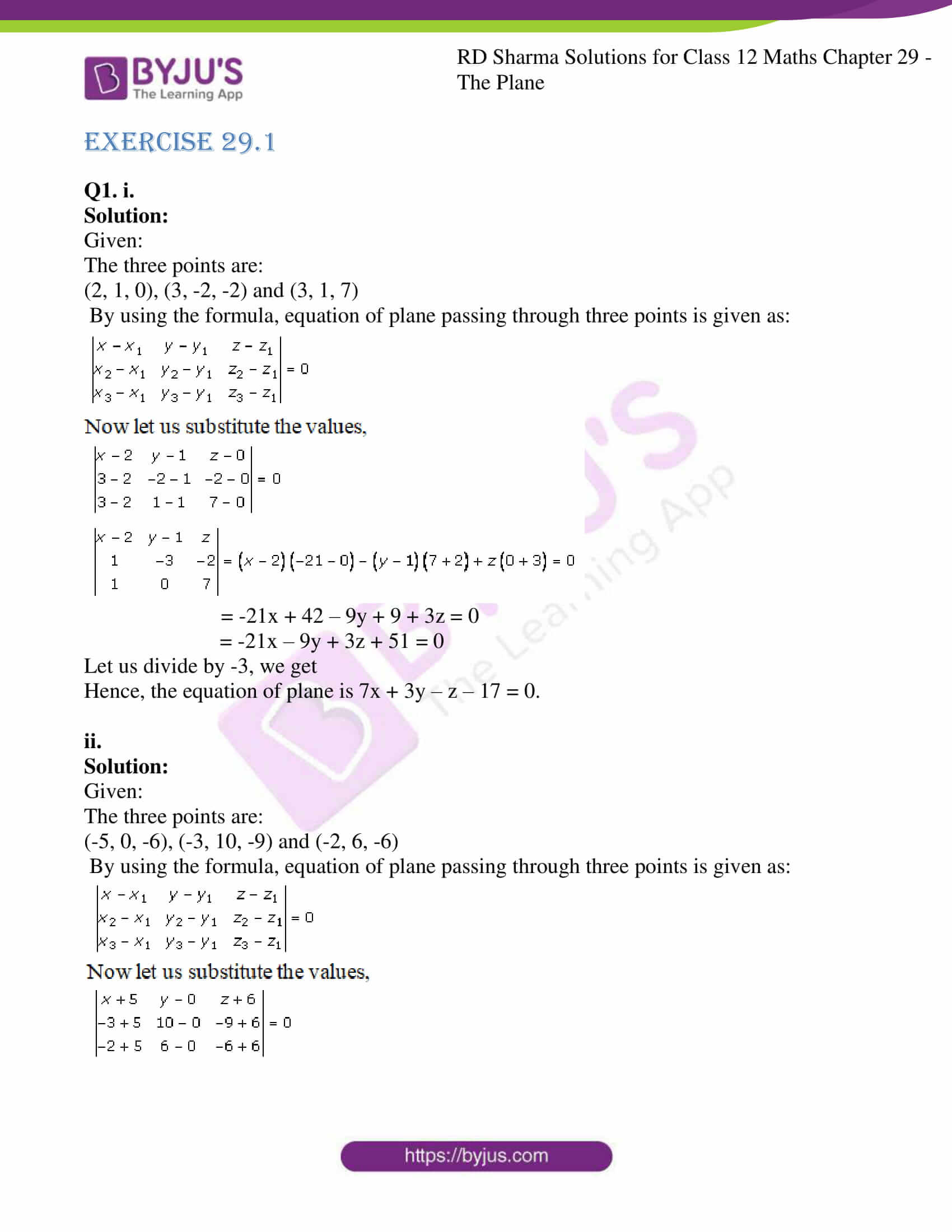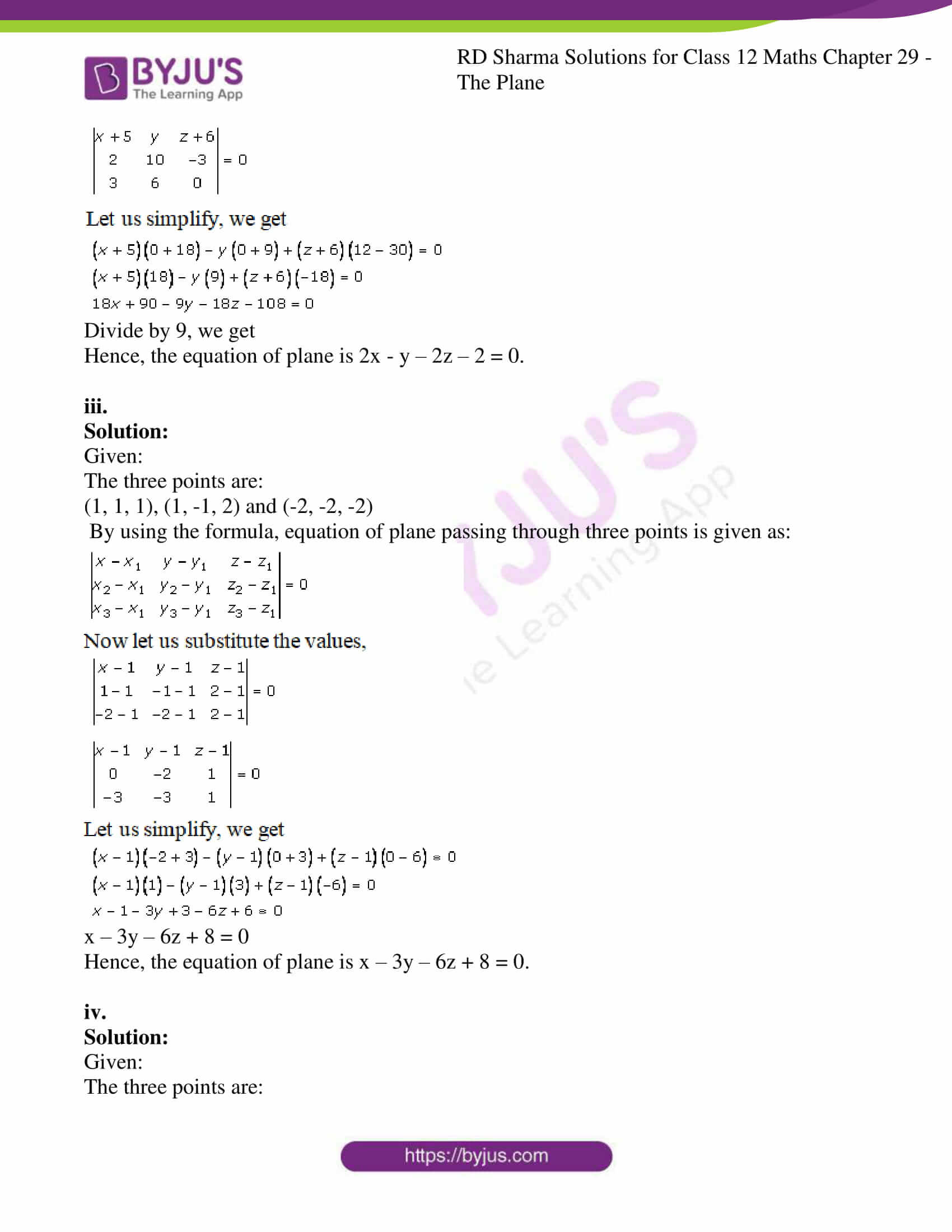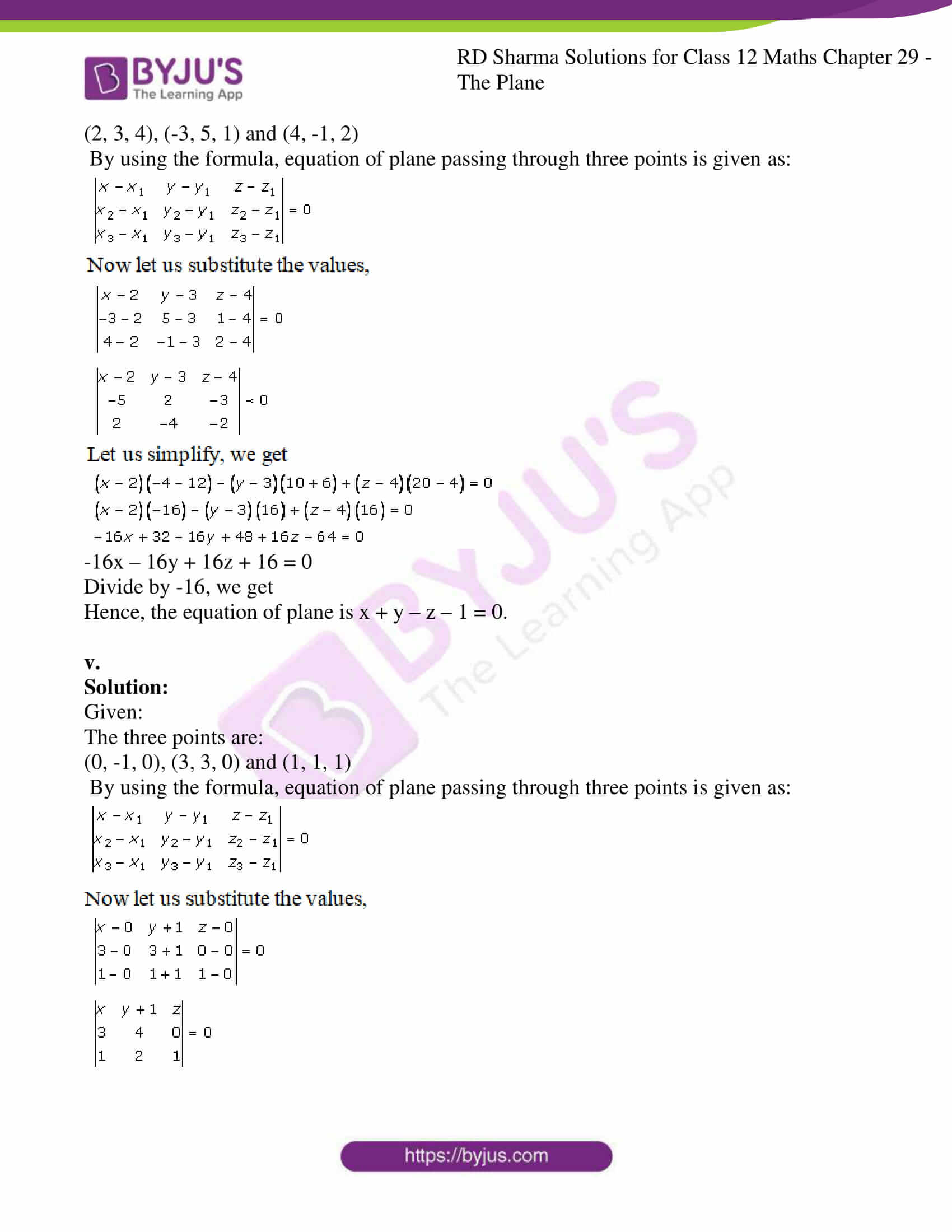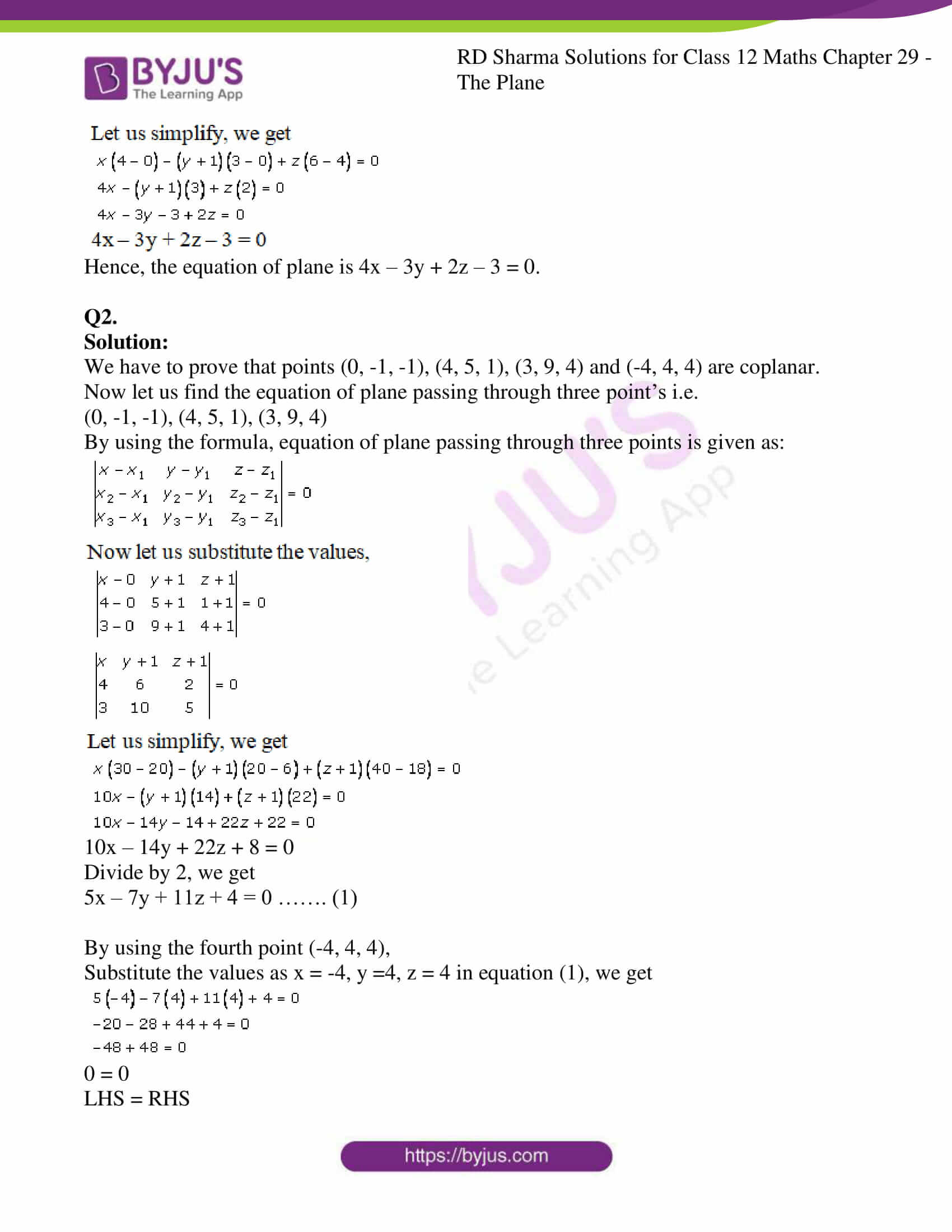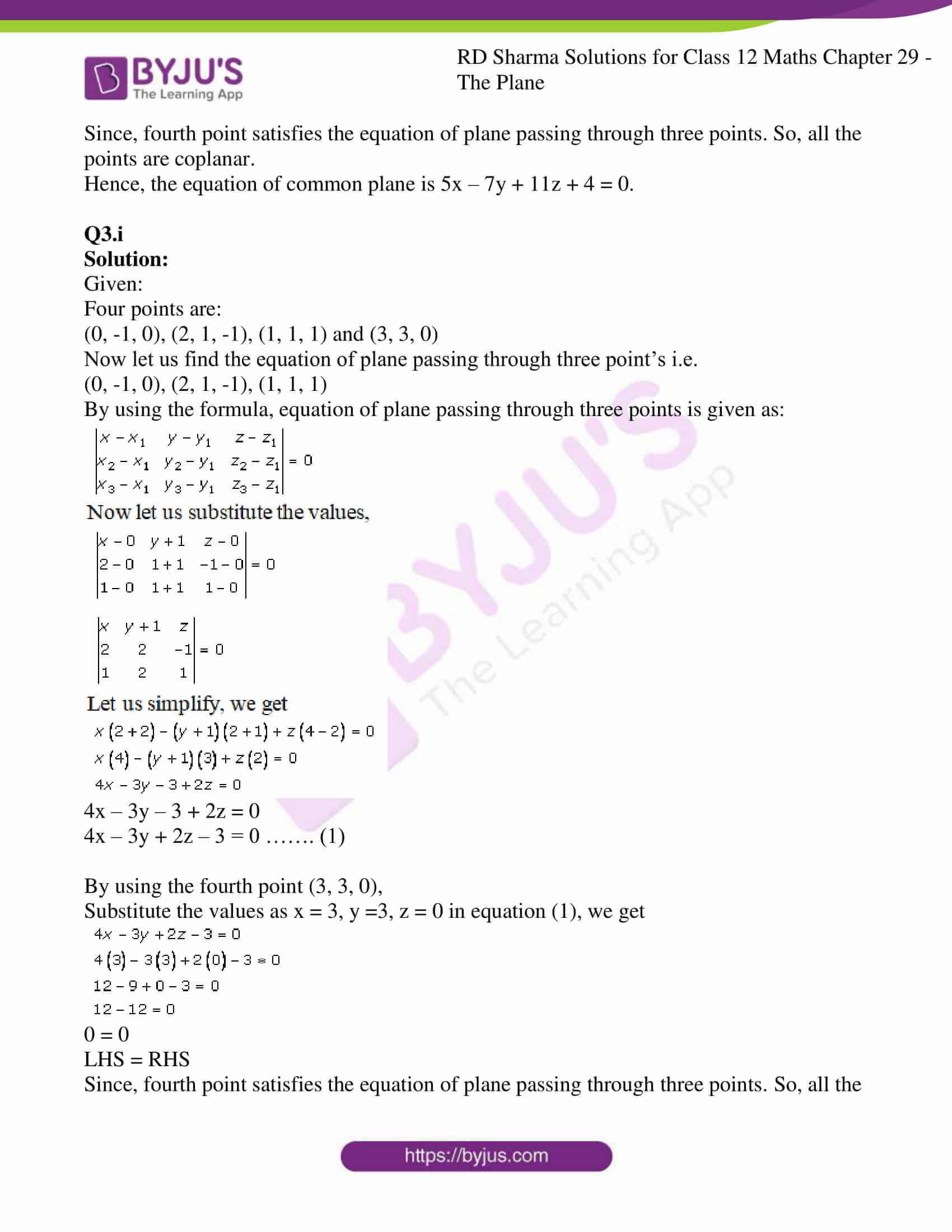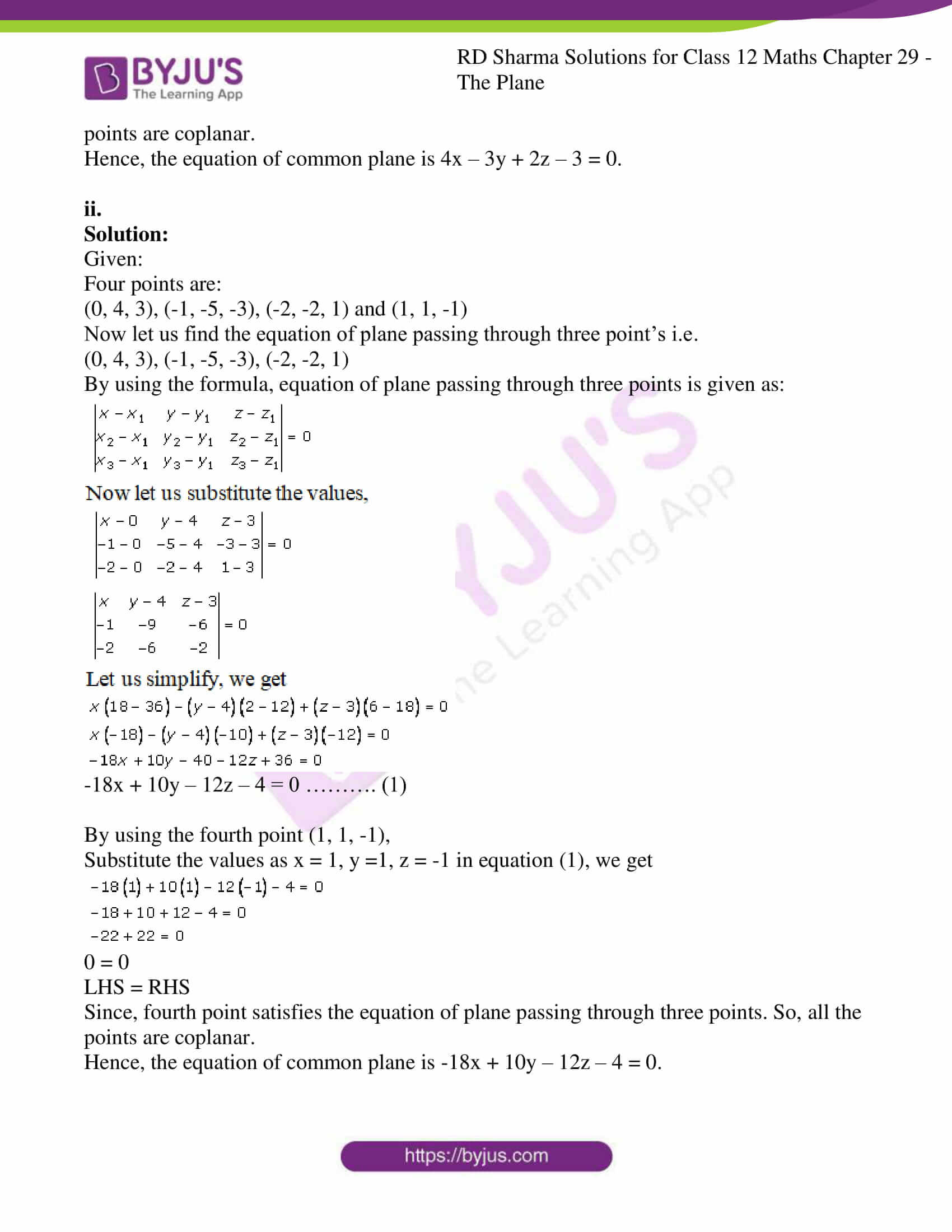### Access RD Sharma Solutions For Class 12 Maths Chapter 29 Exercise 1

EXERCISE 29.1

Q1. i.

Solution:

Given:

The three points are:

(2, 1, 0), (3, -2, -2) and (3, 1, 7)

By using the formula, equation of plane passing through three points is given as: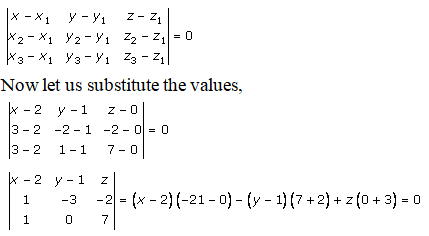= -21x + 42 – 9y + 9 + 3z = 0

= -21x – 9y + 3z + 51 = 0

Let us divide by -3, we get

Hence, the equation of plane is 7x + 3y – z – 17 = 0.

ii.

Solution:

Given:

The three points are:

(-5, 0, -6), (-3, 10, -9) and (-2, 6, -6)

By using the formula, equation of plane passing through three points is given as: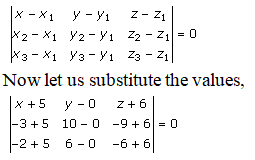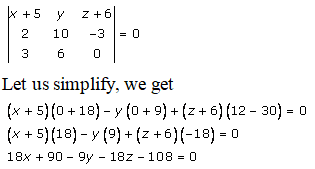Divide by 9, we get

Hence, the equation of plane is 2x – y – 2z – 2 = 0.

iii.

Solution:

Given:

The three points are:

(1, 1, 1), (1, -1, 2) and (-2, -2, -2)

By using the formula, equation of plane passing through three points is given as: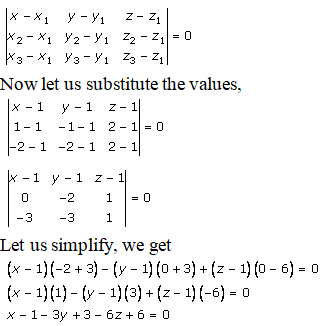x – 3y – 6z + 8 = 0

Hence, the equation of plane is x – 3y – 6z + 8 = 0.

iv.

Solution:

Given:

The three points are:

(2, 3, 4), (-3, 5, 1) and (4, -1, 2)

By using the formula, equation of plane passing through three points is given as: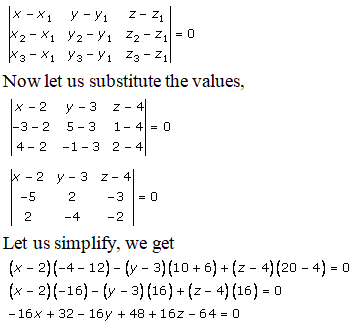-16x – 16y + 16z + 16 = 0

Divide by -16, we get

Hence, the equation of plane is x + y – z – 1 = 0.

v.

Solution:

Given:

The three points are:

(0, -1, 0), (3, 3, 0) and (1, 1, 1)

By using the formula, equation of plane passing through three points is given as: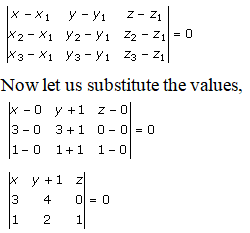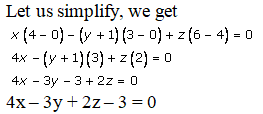Hence, the equation of plane is 4x – 3y + 2z – 3 = 0.

Q2.

Solution:

We have to prove that points (0, -1, -1), (4, 5, 1), (3, 9, 4) and (-4, 4, 4) are coplanar.

Now let us find the equation of plane passing through three point’s i.e.

(0, -1, -1), (4, 5, 1), (3, 9, 4)

By using the formula, equation of plane passing through three points is given as: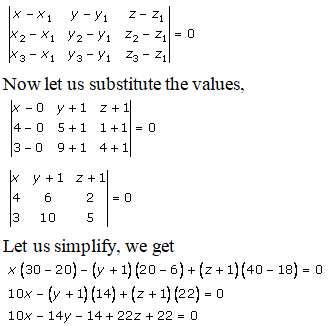10x – 14y + 22z + 8 = 0

Divide by 2, we get

5x – 7y + 11z + 4 = 0 ……. (1)

By using the fourth point (-4, 4, 4),

Substitute the values as x = -4, y =4, z = 4 in equation (1), we get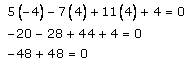0 = 0

LHS = RHS

Since, fourth point satisfies the equation of plane passing through three points. So, all the points are coplanar.

Hence, the equation of common plane is 5x – 7y + 11z + 4 = 0.

Q3.i

Solution:

Given:

Four points are:

(0, -1, 0), (2, 1, -1), (1, 1, 1) and (3, 3, 0)

Now let us find the equation of plane passing through three point’s i.e.

(0, -1, 0), (2, 1, -1), (1, 1, 1)

By using the formula, equation of plane passing through three points is given as: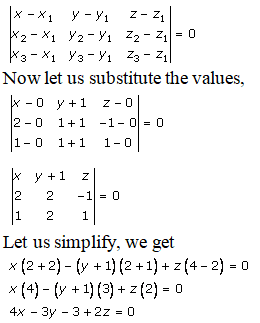4x – 3y – 3 + 2z = 0

4x – 3y + 2z – 3 = 0 ……. (1)

By using the fourth point (3, 3, 0),

Substitute the values as x = 3, y =3, z = 0 in equation (1), we get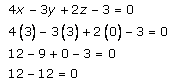0 = 0

LHS = RHS

Since, fourth point satisfies the equation of plane passing through three points. So, all the points are coplanar.

Hence, the equation of common plane is 4x – 3y + 2z – 3 = 0.

ii.

Solution:

Given:

Four points are:

(0, 4, 3), (-1, -5, -3), (-2, -2, 1) and (1, 1, -1)

Now let us find the equation of plane passing through three point’s i.e.

(0, 4, 3), (-1, -5, -3), (-2, -2, 1)

By using the formula, equation of plane passing through three points is given as: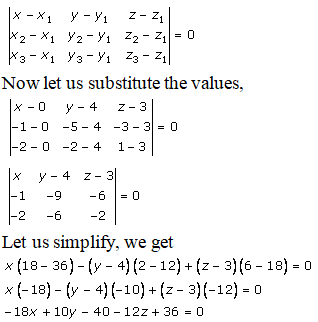-18x + 10y – 12z – 4 = 0 ………. (1)

By using the fourth point (1, 1, -1),

Substitute the values as x = 1, y =1, z = -1 in equation (1), we get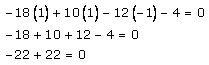0 = 0

LHS = RHS

Since, fourth point satisfies the equation of plane passing through three points. So, all the points are coplanar.

Hence, the equation of common plane is -18x + 10y – 12z – 4 = 0.